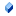This documentation is for the old version. Go to the latest Graphics Mill docs

# Accessing Pixel Data

Graphics Mill for .NET provides a wide range of methods to manipulate images—transformations, effects, conversion between pixel formats, etc. However you may want to implement some custom algorithms. In this case you need to get an access to the bitmap pixels.

There are two ways of accessing the pixels with Graphics Mill for .NET: high-level access and low-level access.

## High-Level Access

This is an access through the GetPixel(Int32, Int32) and SetPixel(Int32, Int32, Color) methods of the Bitmap class. These methods have X and Y parameters which specify the coordinates of the pixel you want to get or set.

The advantage of using a high-level access is that it provides run-time boundary check, so you will never get an access violation problem if you specify wrong coordinates. Also, you don't need to know the physical structure of the pixel. However, it has a weakness—too low performance. So we do not recommend using it for intensive pixel processing. But if you need to simply change a color of several points, this method is very convenient.

## Low-Level Access

This is a direct access to pixel data. Let's examine how to do it.

### How To Access Pixels Directly

Working with pixels at the low level in the Graphics Mill for .NET is similar to the low-level access to pixels in the GDI+. In general, you will perform the following actions.

1. Lock the memory region that contains pixel data using the LockBits method of the Bitmap class. It will return an instance of the BitmapData class.
2. Get a pointer to the data via the Scan0 property of BitmapData class. This value points at the beginning of the block of memory where pixels are stored.
3. Get a number of bytes which are stored in a single row of the bitmap using the Stride property.
4. Get a number of bits which are used to represent a single pixel via the BitsPerPixel property.

Now, you have all means to process pixels directly.

After you are done, unlock the BitmapData using the UnlockBits(BitmapData) method of the Bitmap class.

To implement an algorithm which works directly with pixels, it is important to know how pixels are stored in memory. Let's examine this.

### How Pixels Are Stored in Memory

Pixel data in Graphics Mill for .NET are stored linearly. It means that the entire bitmap occupies a contiguous memory area. All rows of pixels are stored one after another: the second row is after the first one, the third—after the second and so on.

Each row contains the same number of bytes. The Scan0 property returns the pointer to the first pixel of the first row (the beginning of the memory occupied by the bitmap). So, to iterate all pixels in the bitmap we need to start from Scan0 and increment the pointer by the BitsPerPixel bits (to get the number of bytes, just divide it by 8). The interpretation of the pixel depends on the PixelFormat property; the meaning of this property is described in the table below. If you need to reach the next row, use the Stride property. It specifies how many bytes are taken by one row. So if you need to get a pixel which is in the same position as the current one, but in the following row, just add the value stored in the Stride property.

The generic formula of getting a pixel in the row i and the column j is the following:

```result = Scan0 + Stride * i + j * BitsPerPixel / 8 ```

Note

This formula is valid only if the pixel size is divisible by 8 (byte size). If, for example, pixel size is 1 or 4 bits, you need to perform special actions to select the appropriate pixel.

The table below demonstrates the order of pixel components. The square brackets specify the content of one byte, bits in bytes are separated by comma. Bytes are separated by a vertical line. The color (and color name) specifies how the pixel part is interpreted.

Member Name Memory OrderFormat1bppIndexed [pixel1,pixel2,pixel3,pixel4,pixel5,pixel6,pixel7,pixel8]Format4bppIndexed [pixel1,pixel2]|[pixel3,pixel4]Format8bppIndexed [pixel1]Format16bppArgb1555 [Alpha,Red,Red,Red,Red,Red,Green,Green]|[Green,Green,Green Red,Red,Red,Red,Red]Format16bppGrayScale [Grayscale]|[Grayscale]Format16bppRgb555 [Not Used,Red,Red,Red,Red,Red,Green,Green]|[Green,Green,Green Blue,Blue,Blue,Blue,Blue]Format16bppRgb565 [Red,Red,Red,Red,Red,Green,Green,Green]|[Green,Green,Green Blue,Blue,Blue,Blue,Blue]Format24bppRgb [Blue]|[Green]|[Red]Format32bppRgb [Blue]|[Green]|[Red]|[Not Used]Format32bppArgb [Blue]|[Green]|[Red]|[Alpha]Format32bppPArgb [Blue]|[Green]|[Red]|[Alpha]Format48bppRgb [Blue]|[Blue]|[Green]|[Green]|[Red]|[Red]Format64bppArgb [Blue]|[Blue]|[Green]|[Green]|[Red]|[Red]|[Alpha]|[Alpha]Format64bppPArgb [Blue]|[Blue]|[Green]|[Green]|[Red]|[Red]|[Alpha]|[Alpha]Format32bppCmyk [Black]|[Yellow]|[Magenta]|[Cyan]Format40bppAcmyk [Black]|[Yellow]|[Magenta]|[Cyan]|[Alpha]Format8bppGrayScale [Grayscale]Format16bppAlphaGrayScale [Grayscale]|[Alpha]Format32bppAlphaGrayScale [Grayscale]|[Grayscale]|[Alpha]|[Alpha]Format64bppCmyk [Black]|[Black]|[Yellow]|[Yellow]|[Magenta]|[Magenta]|[Cyan]|[Cyan]Format80bppAcmyk [Black]|[Black]|[Yellow]|[Yellow]|[Magenta]|[Magenta]|[Cyan]|[Cyan]|[Alpha]|[Alpha]

### Code Example

Now, let us see an example of code snippet which works with pixels directly. Let it be a bitmap invertion (image negative).

Visual Basic
```Dim bitmap As New Bitmap
Dim data As BitmapData = bitmap.LockBits()

'How many bytes one pixel occupies
Dim pixelSize As Integer = data.BitsPerPixel / 8
'Number of bytes in a row
Dim stride As Integer = data.Stride
'Number of rows
Dim height As Integer = data.Height
'A pointer to the beginning of the pixel data region
Dim pointer As IntPtr = data.Scan0
'An array representing a single pixel
Dim pixel(pixelSize - 1) As Byte

Dim i, j, k As Integer
For i = 0 To height - 1
j = 0
Do While j < stride

Marshal.Copy(pointer, pixel, 0, pixelSize)

'Now we can modify the pixel, for example, invert it
For k = 0 To 3
pixel(k) = 255 - pixel(k)
Next k

'...and write it back into the bitmap
Marshal.Copy(pixel, 0, pointer, pixelSize)

pointer = IntPtr.op_Explicit(pointer.ToInt32() + pixelSize)
j = j + pixelSize
Loop
Next i

bitmap.UnlockBits(data)
bitmap.Save("D:\image-32bppARGB-inverted.png")
bitmap.Dispose()
```
C#
```Bitmap bitmap = new Bitmap();
BitmapData data = bitmap.LockBits();

unsafe
{
//A pointer to the beginning of the pixel data region
byte* pointer = (byte*)(data.Scan0.ToPointer());
//Number of bytes in a row
int stride = data.Stride;
//Number of rows
int height = data.Height;

byte* position;

for (int i = 0; i < height; i++)
{
position = pointer + stride * i;
for (int j = 0; j < stride; j++)
{
//Now we can modify the pixel, for example, invert it
*position = (byte)(255 - *position);
position++;
}
}
}

bitmap.UnlockBits(data);
bitmap.Save(@"D:\image-32bppARGB-inverted.png");
bitmap.Dispose();
```